# Part 8: Similarity

Are you unsure about the differences between proving congruent and similar triangles? Well, don't worry! In this guide, we will explain everything about similar triangles. We will go through the meaning of similarity, the scale factors and the four tests of similarities in detail.## What’s similarity and why is it important?

Assessing the similarity of shapes is a vital part of geometry, which you will continue to use in mathematics in later years.

However many students find it confusing.

If you could do with brushing up, or even if you’re learning this for the first time, read on to see our tips and tricks for working with similarity problems.

## NSW Syllabus outcomes

 Syllabus outcome Explanation Use the enlargement transformations to explain similarity and to develop the conditions for triangles to be similar (ACMMG220)Investigate the minimum conditions needed, and establish the four tests, for two triangles to be similarDetermine whether two triangles are similar using an appropriate test This means that we will understand what it means for two shapes to be called similar, and how we can prove that two triangles are similar. Apply logical reasoning, including the use of congruence and similarity, to proofs and numerical exercises involving plane shapes (ACMMG244)Write formal proofs of the similarity of triangles in the standard four- or five-line format, preserving the matching order of vertices, identifying the scale factor when appropriate, and drawing relevant conclusions from this similarityEstablish and apply for two similar figures with similarity ratio $$1:k$$ the ratios of angles, intervals, areas and volumes. This means that we will learn how to write a proof that two triangles are similar, and what that means for their angles, side lengths, areas and volumes.

## Assumed knowledge

• Congruency
• Ratios
• Angles on parallel lines

## Test your knowledge of Congruency before we get started!## What does similarity mean?

Similarity arises when shapes are resized, rotated or reflected.

In principle, similar objects must have the same proportions in their shape and size.

They have the same shape, but not necessarily the same size.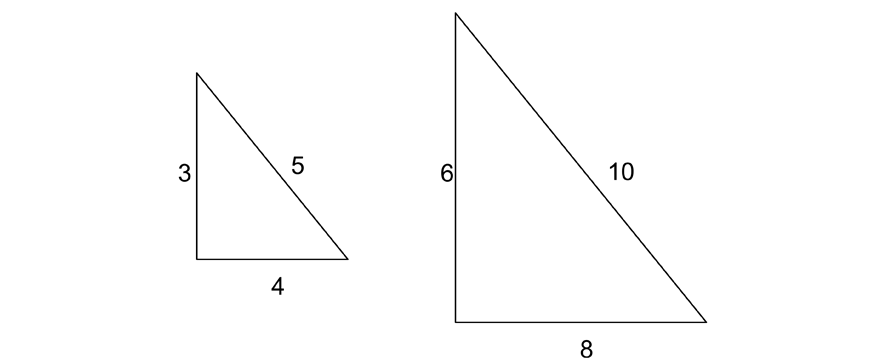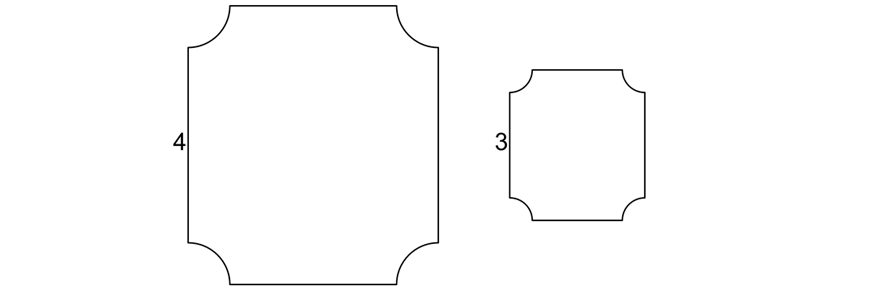For example, when ordering a pizza, there might be small and large size options. Both sizes of the pizza will be “similar” as they are the same, just resized.

Other examples of similar figures include scale models, where the scale model imitates the real-life object, but in much smaller proportions.

Note: congruent figures are similar, but not all similar figures are congruent!

## What is the scale factor?

A figure and its enlargement are called similar figures.

In similar figures, all corresponding sides are in ratio.

This ratio is called the scale factor.

Also, all corresponding angles are equal.

### Example

What is the scale factor for these triangles?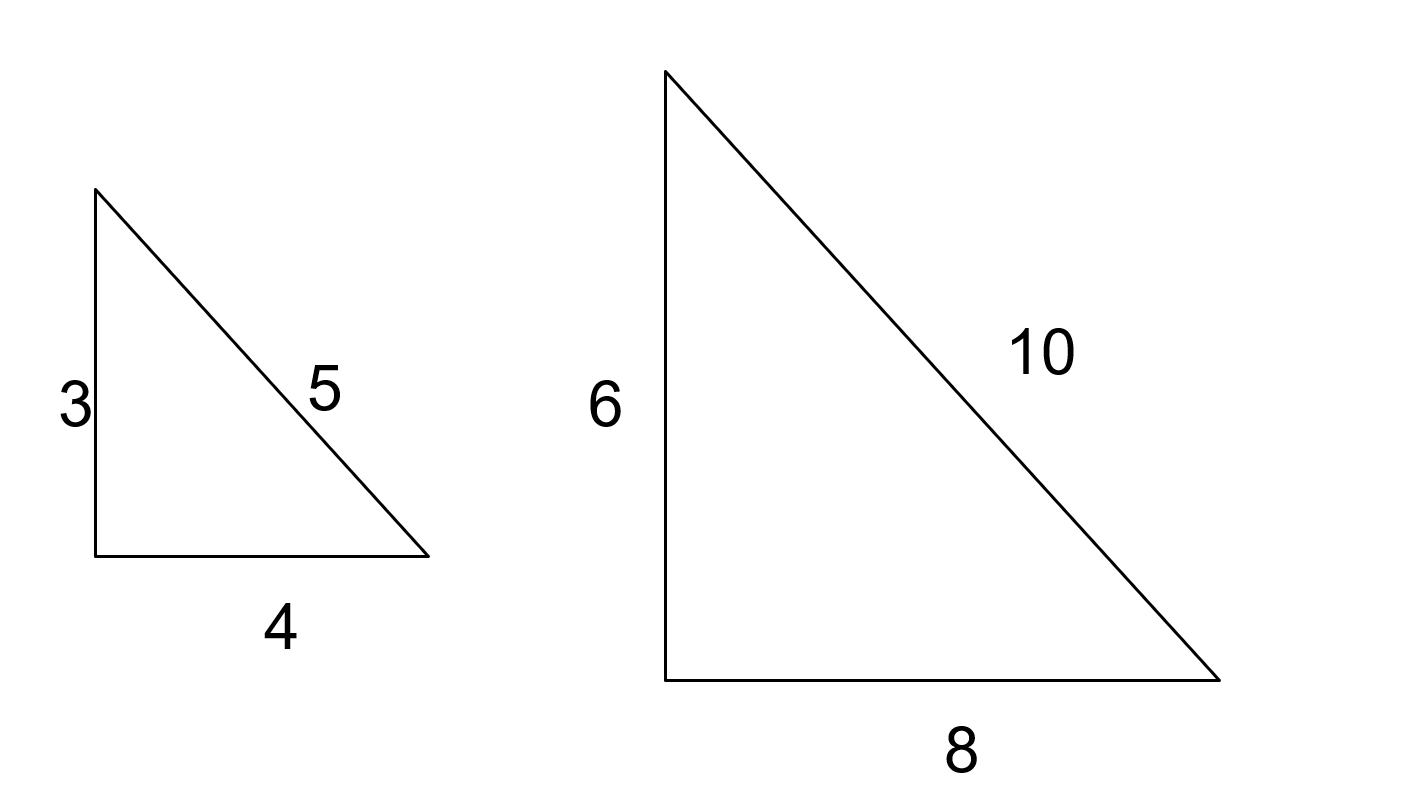Solution:

Notice that the corresponding side lengths between the two triangles share the same ratio.

This ratio is $$6:3 = 8:4 = 10:5$$, the scale factor is therefore $$2:1$$.

This is an enlargement factor.

Alternatively, the factor can be thought of as $$1:2$$ from the larger triangle’s perspective.

In this case, it is a reduction factor.

If all corresponding sides of two shapes are enlarged/reduced by the same ratio, they are similar shapes.

You will need to be able to identify these situations.

The mathematical symbol for “is similar to” is |||.

In the diagram below, $$\triangle ABC \ ||| \ \triangle DEF$$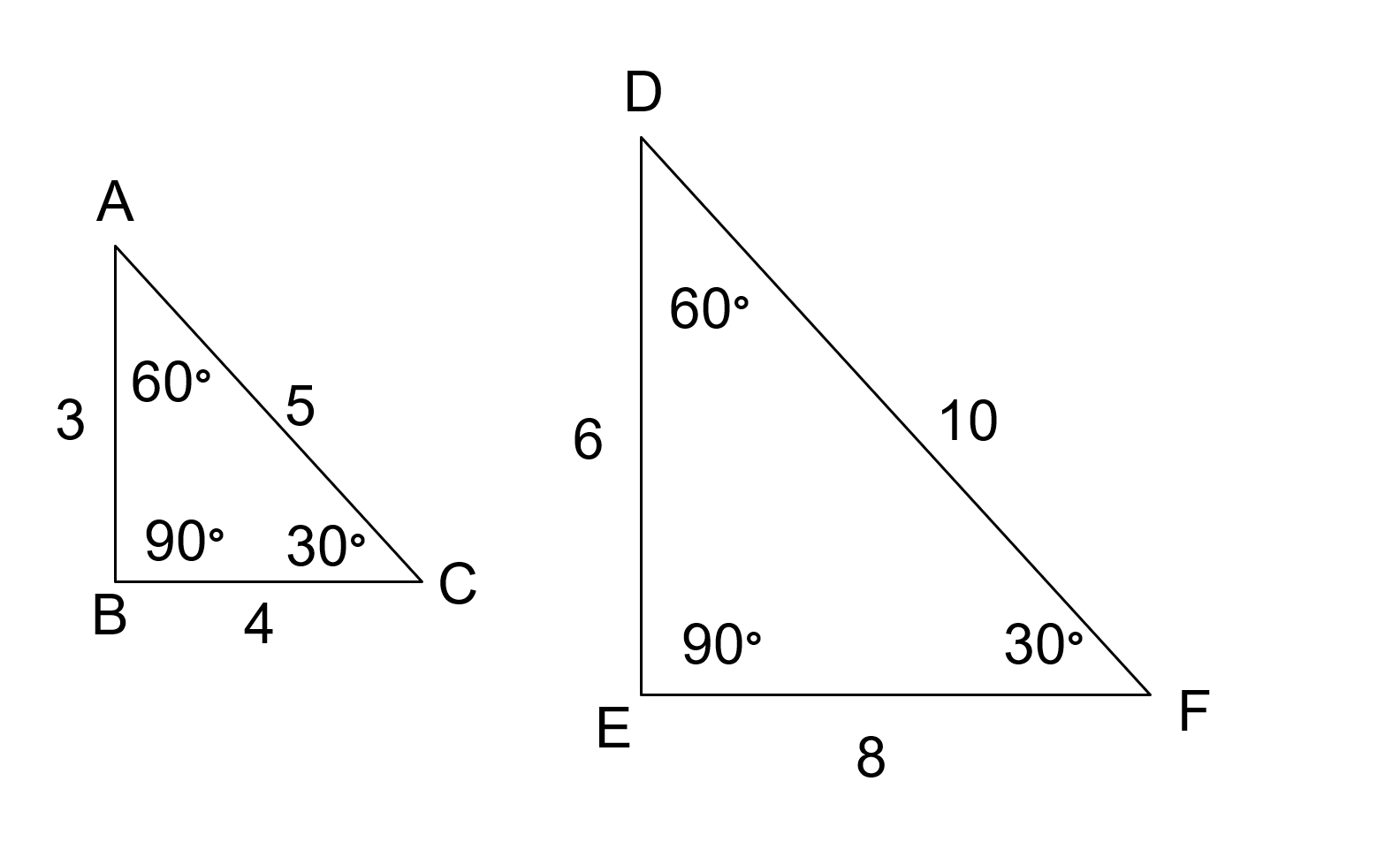This means that

• $$\angle A = \angle D, \angle B = \angle E, \angle C = \angle F \ \text{(similar figured have the same angles)}$$
• $$\frac{AB}{DE} = \frac{BC}{ED} = \frac{AC}{DF} = \frac{1}{2} \ \text{(similar figures have side lengths in ratio)}$$

### What is the effect of the scale factor on the perimeters and areas of the similar figures?

Let’s investigate the same similar triangles as above.

The perimeter of $$\triangle ABC$$ is $$3 + 4 + 5 = 12$$ units.

Its area is $$\frac{1}{2} \times 3 \times 4 = 6$$ units squared.

The perimeter of  $$\triangle DEF = 24$$ units.

Its area is $$\frac{1}{2} \times 6 \times 8 = 24$$ units squared.

We can see that the ratio of the perimeters is the same as the scale factor.

The ratio of areas is $$1:4$$, which is $$1:2$$.

If the scale factor for similar figures is $$a:b$$, the ratio of their areas is the scale factor squared: $$a^2 : b^2$$.

Learn about Similarity and other Year 8 Maths topics before it’s taught in school! Learn more about our Year 8 Maths Term Course.## The four tests of similarity

Like congruency, we have four tests of similarity.

Note: These tests DO NOT have standard abbreviations like the congruency tests.

Check with your school teacher for the best way to notate them.

### Why do I need these 4 tests?

Students need to know the four tests, identify when to apply them, and understand their implications for the scale factor.

Note: matching sides are opposite matching angles

i.e. A side of one triangle matches with the side of another triangle if the two sides are opposite the same angle.

### Test 1: All sides in a ratio

Two triangles are similar when the lengths of the matching sides of the two triangles are in ratio.

Remember, all matching sides have to be in the same ratio for them to be similar.

Example

1. Which of the following pairs of triangles are similar?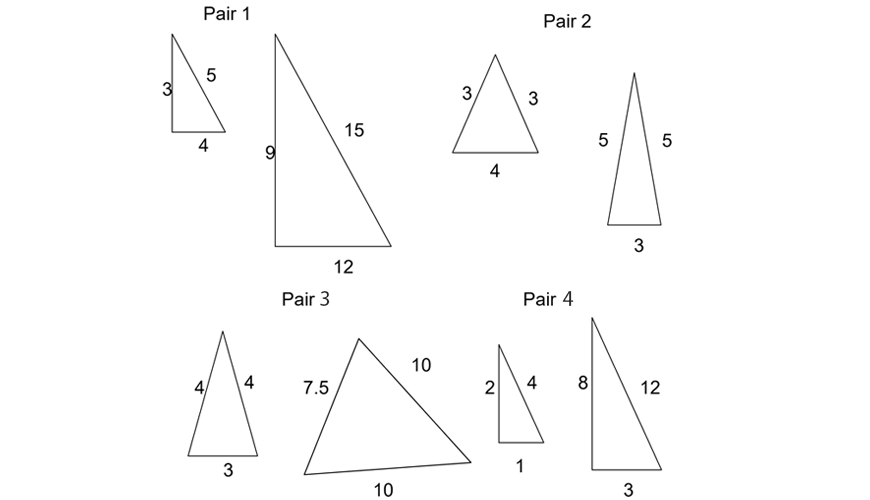Solution:

Pair 1 are similar as we can see that all sides in the triangle on the right are $$3$$ times the size of the corresponding sides on the left.
i.e. the sides are in a ratio of $$1:3$$.

Pair 2 are not similar as we cannot match corresponding sides of the triangles so that all of the sides are in ratio $$(3:5 ≠ 4:3)$$

Pair 3 are similar as matching the equal sides in both triangles gives all of the sides in the same ratio $$(4:10 ≠ 3:7.5)$$

Pair 4 are not similar, even though we can match two sides which are in ratio (), because the remaining pair are not in the same ratio $$(2:4 ≠ 1:3)$$

### Test 2: Equiangular

Two triangles are similar if two angles in one triangle are equal to two angles in the other triangle.

Note: The two angles matching automatically implies the third angle also matches.

Why?

Think of the angle sum of a triangle.

Example

1. Which of the following pairs of triangles are similar? What is the remaining angle in each triangle?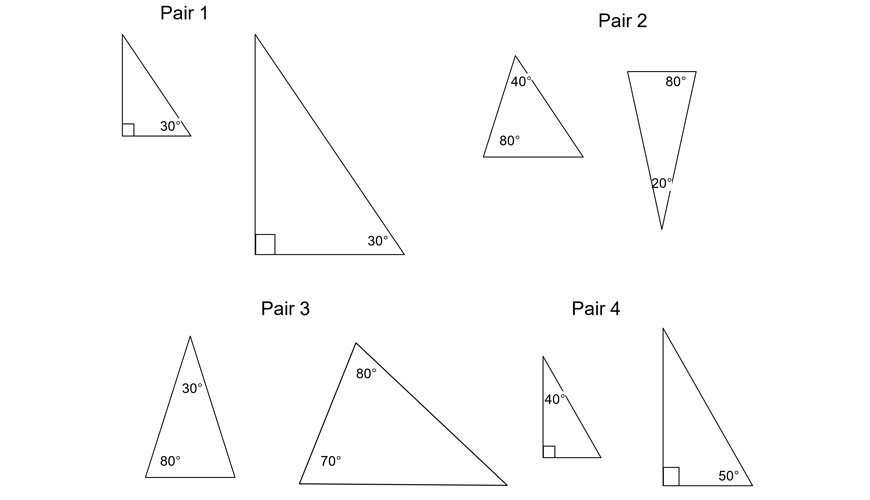Solution:

Pair 1 are similar, as we have angles of  $$90°$$ and  $$30°$$ in both triangles, giving us two equal angles.

Using the angle sum of a triangle, we can determine that the unlabeled angle in both triangles is $$180° = (90°+30°) = 60°$$.

Pair 2 are not similar, despite the fact that we have $$80°$$ in both triangles, as the remaining angles ($$40°$$ and $$20°$$, respectively) are not equal.

We can again use angle sums of triangles to determine that the unlabeled angle in the left triangle is $$60°$$, and the unlabeled angle in the right triangle is $$80°$$.

Pair 3 and pair 4 appear to not be similar at first glance since neither have 2 pairs of labelled matching equal angles.

However, in both cases, when we work out the unlabeled angle for one of the triangles we see that it is equal to the labelled angle in the other.

i.e. for the right triangle in pair $$3$$ the unlabeled angle is $$30°$$.

And for the right triangle in pair $$4$$ the unlabeled angle is $$40°$$.

This means that in both pairs of triangles, we can find $$2$$ pairs of equal angles.

So, pair $$3$$ and pair $$4$$ are both similar.

### Test 3: Two sides in ratio and included angle equal

Two triangles are similar when two pairs of matching sides are in ratio and their included angle is equal.

Example

1. Which of the following pairs of triangles are similar?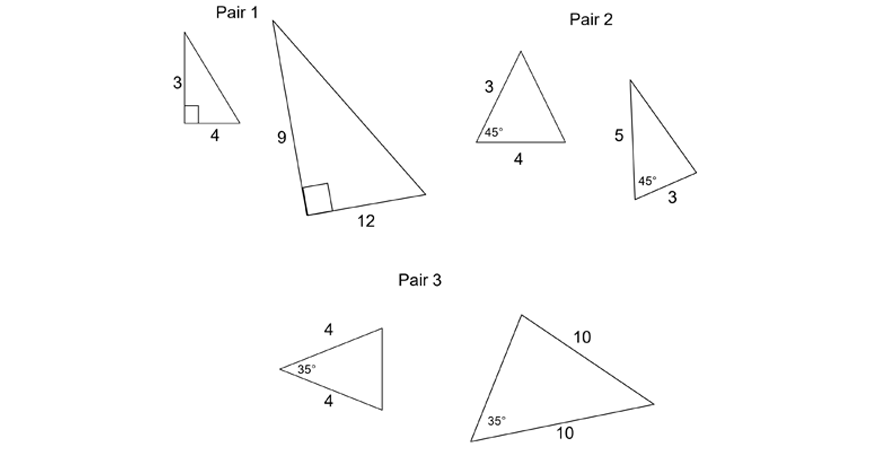Solution:

Pair 1 are similar as there are two sides in ratio ($$3:9 = 4:12$$, ratio is $$1:3$$ in simplest form) and the included angle is equal.

i.e. The angle between the two sides in ratio is $$90°$$ in both triangles.

Pair 2 are not similar as the two labelled sides on both triangles are not in ratio, as $$4:5 ≠ 3:3$$ (and $$3:5 ≠ 4:3$$).

We do have the included angle in both triangles as $$45°$$, but this is not enough.

Pair 3 are not similar even though we have two sides in ratio ($$4:10, 2:5$$ in simplest form), and an angle of $$35°$$ in both triangles.

This is because the equal angle is not the included angle.

I.e. It is not between the two sides which are in ratio.

### Test 4: Hypotenuse and pair of sides in ratio

Two right-angled triangles are similar when their hypotenuses and another pair of matching sides are in the same ratio.

Example

1. Which of the following pairs of triangles are similar?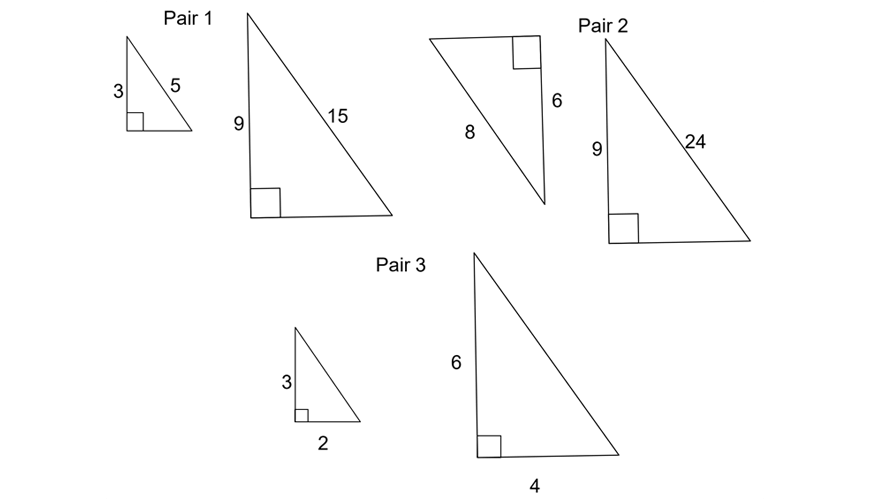Solution:

Pair 1 are similar, as these are right-angles triangles and, we can see that the hypotenuses and one other matching pair of sides of the triangles are both in a ratio of $$1:3$$.

Pair 2 are not similar, despite being right-angled triangles.

The ratio between the hypotenuses ($$8:24$$, or $$1:3$$ in simplest form) is not the same as the ratio between the other pair of matching sides ($$6:9$$, or $$2:3$$ in simplest form.)

Pair 3 are in fact similar. However, there is a catch.

This pair is not an example of test 4, since the hypotenuses are not labelled. This means that we do not know their ratio.

However, the right angle is in fact the included angle between the two labelled sides… which are in a ratio of $$2:4, 3:6$$, or $$1:2$$ in simplest form.

This means that the easiest way to tell these triangles are similar is using test 3.

Note: we could work out the hypotenuses using Pythagoras’ Theorem and then use test 4, but it is simpler to just use test 3.

You may notice ‘similarity’ with these tests and the congruency tests.

A reminder that proving two triangles are congruent implies they’re similar as well, but not necessarily vice versa!

Now try these checkpoint questions for practice!

## Checkpoint questions

1. What is the scale factor between these two congruent triangles?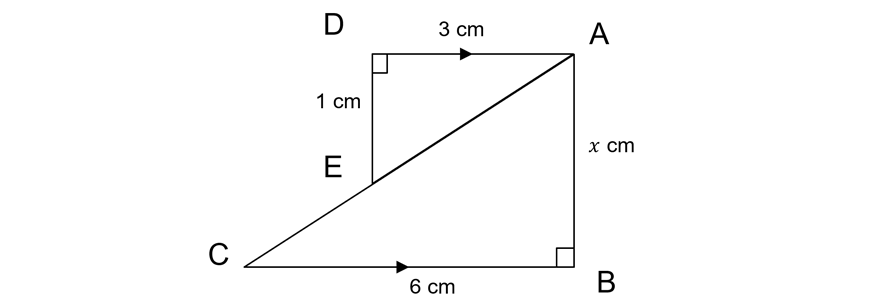2. Prove that $$\triangle ABC$$ and $$\triangle ADE$$ are similar (Hint: remember parallel lines results.) Hence find the value of $$x$$.

3. Prove the triangles are similar. The area of the larger triangle is $$8 cm^2$$. Hence find the area of the smaller triangle.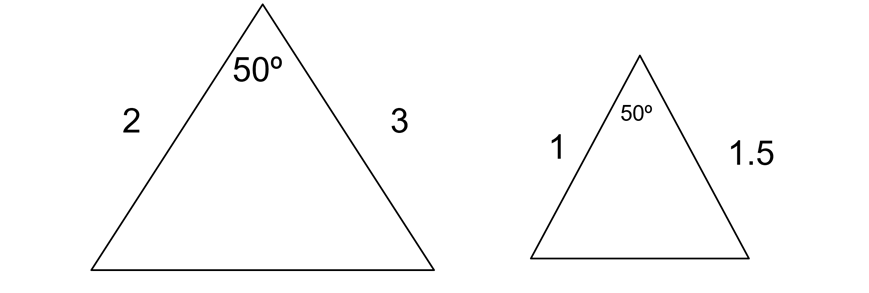4. Prove the triangles are similar. What is the scale factor between them? What is the ratio of their perimeters?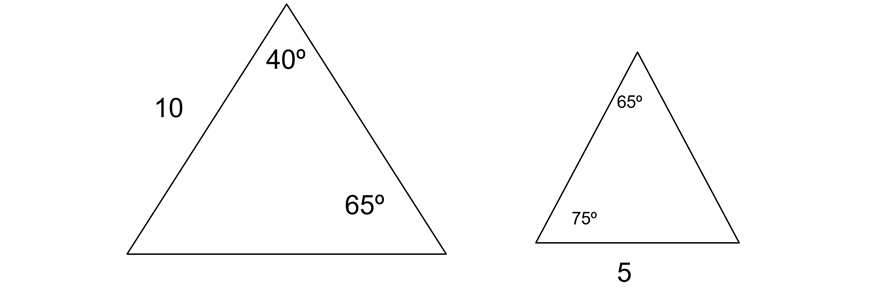5. Show that the following two triangles $$( \triangle ABC \text{and} \triangle ADE)$$ are similar: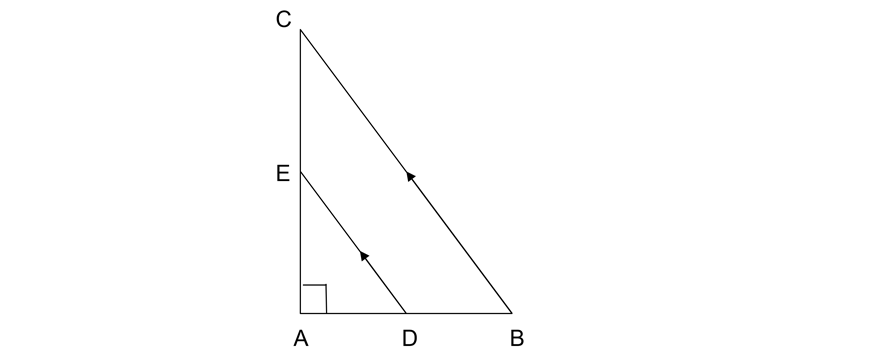6. In the diagram below, $$BE = CF$$. Show that $$\triangle AEB \text{and} \triangle AGH$$ are similar.

Assume that $$\triangle DFC$$ and $$\triangle DIJ$$ can be shown to be similar also. What is the length $$JH$$?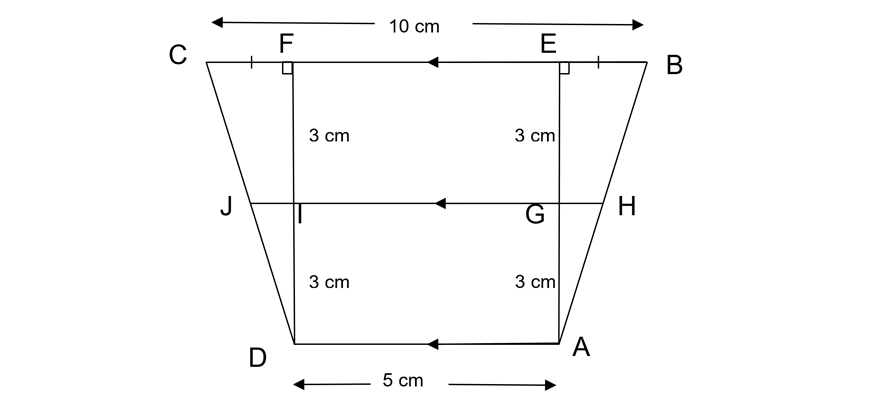7. A conical flask has height $$7 \ cm$$ (flask shaped like a cone).

Water is poured in such that the radius of the water is $$3 \ cm$$ and the height within the flask is $$3.5 \ cm$$. Find the radius of the conical flask.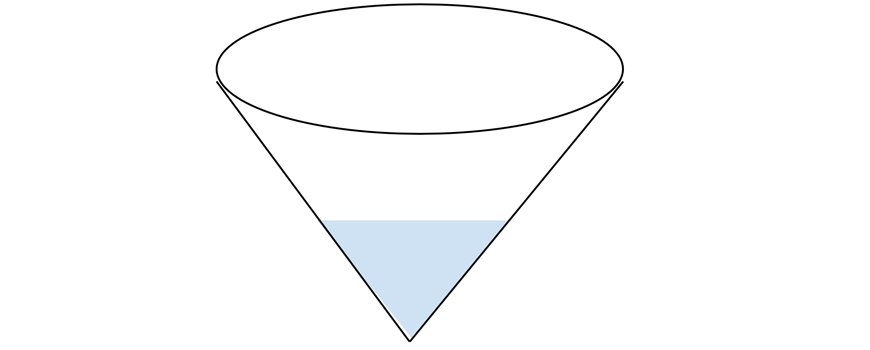8. State why $$\triangle ABC ||| \triangle CDE$$.

Find $$DE$$. Hence find $$AB$$.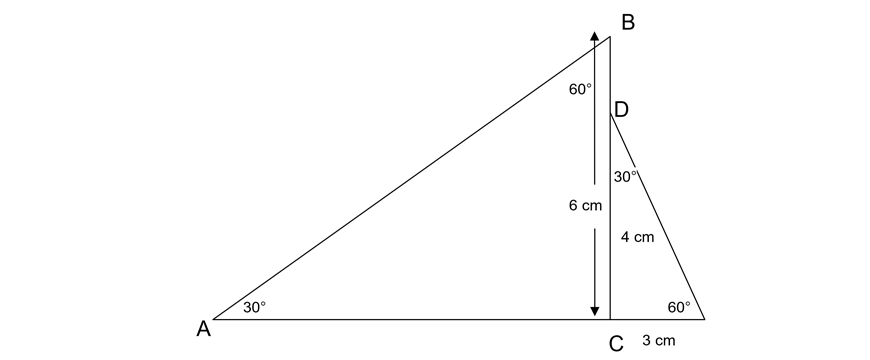9. Below are two regular hexagons. State why they’re similar.

What is the ratio of the area of the smaller hexagon to the area of the larger hexagon?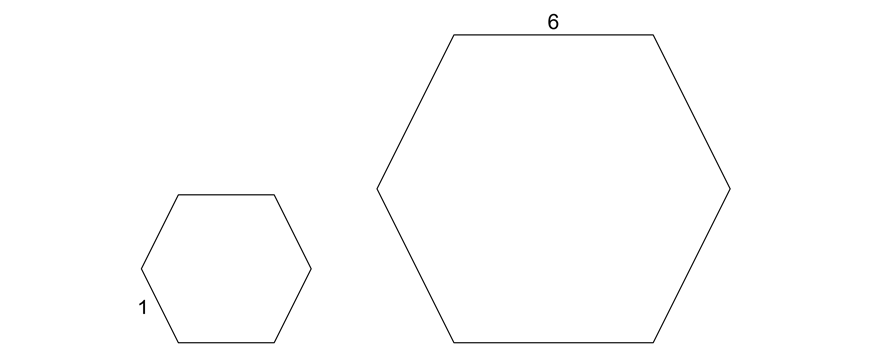10. This is a photo of the Eiffel Tower. A photographer used Photoshop to make some effects and edits. The photographer zoomed in on the photo (see image on the right).

He found that the Eiffel Tower now took up $$4$$ times more area on his screen. How much did he zoom in by?

## Solutions

1. $$1:1$$. Congruent triangles are similar but also have exactly the same size.

2. Proving similarity often relies on observing equal angles.

We notice that:

\begin{align*}
\angle ABC &= \angle ADE = 90° \ (\text{given}) \\
\angle DAE &= \angle ACB \ (\text{(Alternate angles are equal}, DA || CB) \\
∴ \triangle ABC ||| \triangle ADE \ (\text{equiangular})
\end{align*}

Then, $$\frac{x}{1} = \frac{6}{3}$$ (matching sides of similar triangles are in ratio).

So, $$x = 2$$

3. Both triangles have $$50°$$

\begin{align*}
\frac{2}{1} = \frac{3}{1.5} = 2:1
\end{align*}

∴ The triangles are similar (two sides are in ratio and included angles are equal)

The ratio of sides is $$2:1$$ so the ratio of areas must be $$4:1$$.

So the area of the smaller triangle is $$2 cm^2$$.

4. We notice that they both have an angle of $$65°$$.

We just need one more angle for them to be similar.

The missing angle in the first triangle is $$180° – 40° – 65° = 75°$$.

∴ The triangles are similar (equiangular).

The ratio of sides must be $$10:5$$. The ratio of their perimeters is also $$10:5$$.

5.

\begin{align*}
\angle CAB \ (\text{is common}) \\
\angle EDA &= \angle CBA \ (\text{corresponding angles are equal}, CB || ED) \\
∴ \triangle ABC &||| \triangle ADE \ (\text{equiangular})
\end{align*}

6.

\begin{align*}
\angle AED &= 90° \ \text{is common} \\
\angle AGH &= \angle AEB \ (\text{corresponding angles are equal}, EB || GH) \\
∴ \triangle AEB &||| \triangle AGH \ \text{equiangular} \\
CF &= EB = 2.5 \\
\frac{AG}{AE} &= \frac{GH}{EB} \ \text{and similarly}, \ \frac{DI}{DF} = \frac{JI}{CF}.
\end{align*}

So, $$\frac{3}{3 + 3} = \frac{GH}{2.5}, \ GH = 1.25$$, and similarly, $$JH = 1.25 + 5 + 1.25 = 7.5 \ cm$$

7. It will be more helpful to visualise the cone in 2D like this.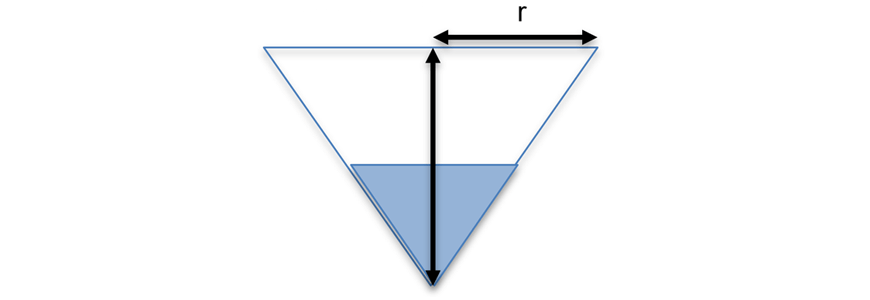So, similar to in Question 5, similar triangles can be shown to have formed.

\begin{align*}
∴ \frac{r}{3} &= \frac{7}{3.5} \ (\text{matching sides of similar triangles are in ratio}) \\
r &= 6 \ cm
\end{align*}

8. The two triangles are equiangular (2 angles are equal), so they must be similar.

So, the ratio of matching sides must be the same, $$\frac{AB}{DE} = \frac{6}{3}$$.

To find $$DE; \ \angle DCE = 180º – 30º – 60º = 90º \ (\text{angle sum of a triangle is} 180º)$$

So, by Pythagoras, $$De^2 = 3^2 + 4^2$$

$$DE = 5 \ cm$$

So, $$AB = 10 \ cm$$

9. They are similar because they’re both regular polygons.

This means all their angles and sides are the same.

Hence, since they’re equiangular and their sides must all be in the same ratio with each other, they’re similar.

The ratio of sides is $$1:6$$.

So, the ratio of the area of the smaller hexagon to the larger hexagon is $$1:36$$.

(Note that the order of the ratio matters! $$1:36 ≠ 36:1$$).

10. By $$2$$ times.

Remember: If the scale factor for similar figures is $$a:b$$, the ratio of their areas is the scale factor squared; $$a^2 : b^2$$

This means if the area was increased by a factor of $$4$$, each side length must’ve been increased by a factor of $$2 (\sqrt4)$$.

Similarity can be thought of what happens when you stretch out an object (keeping the same proportions), like you would when you enlarge a photo (or zooming out!).

© Matrix Education and www.matrix.edu.au, 2021. Unauthorised use and/or duplication of this material without express and written permission from this site’s author and/or owner is strictly prohibited. Excerpts and links may be used, provided that full and clear credit is given to Matrix Education and www.matrix.edu.au with appropriate and specific direction to the original content.